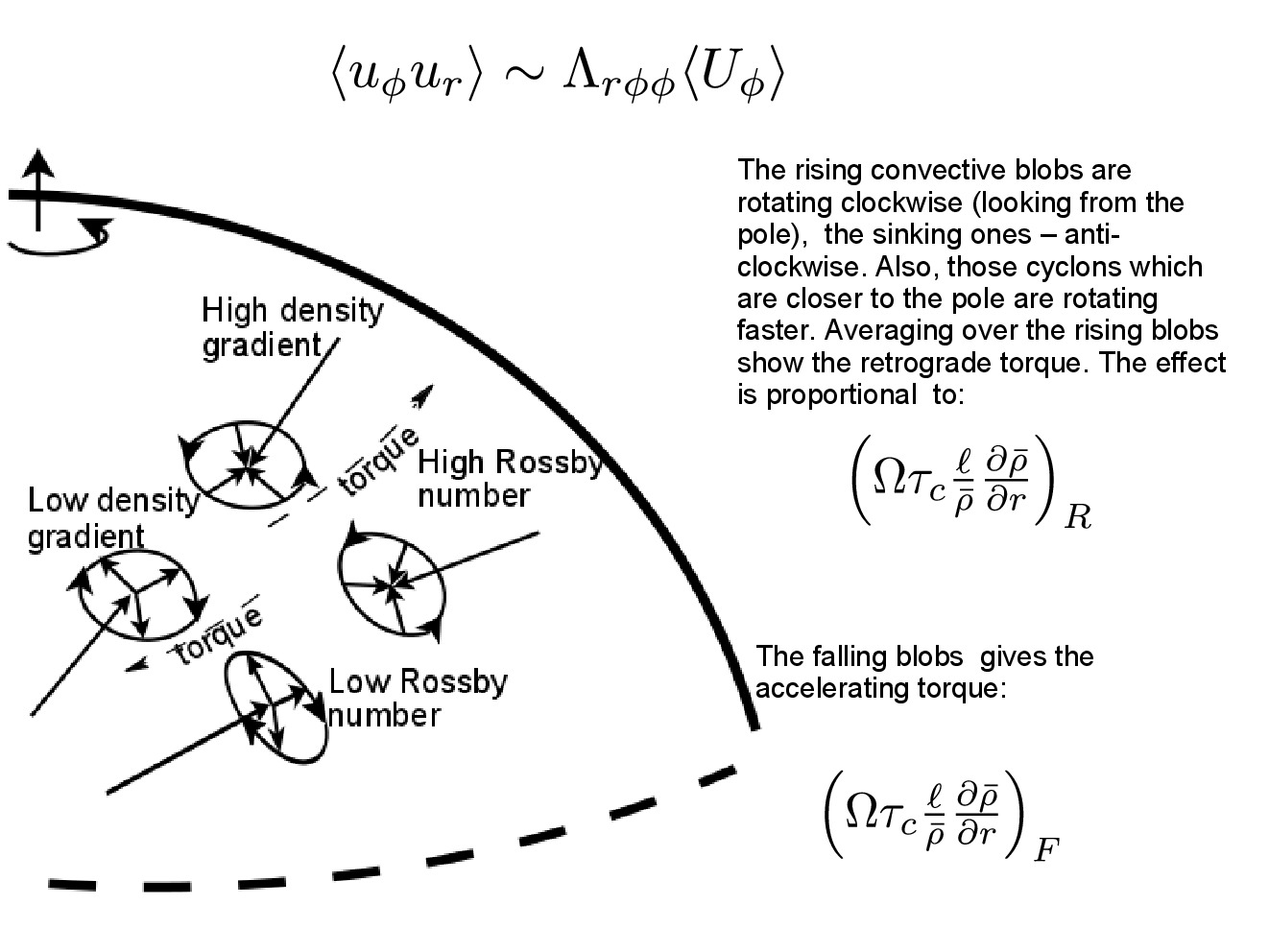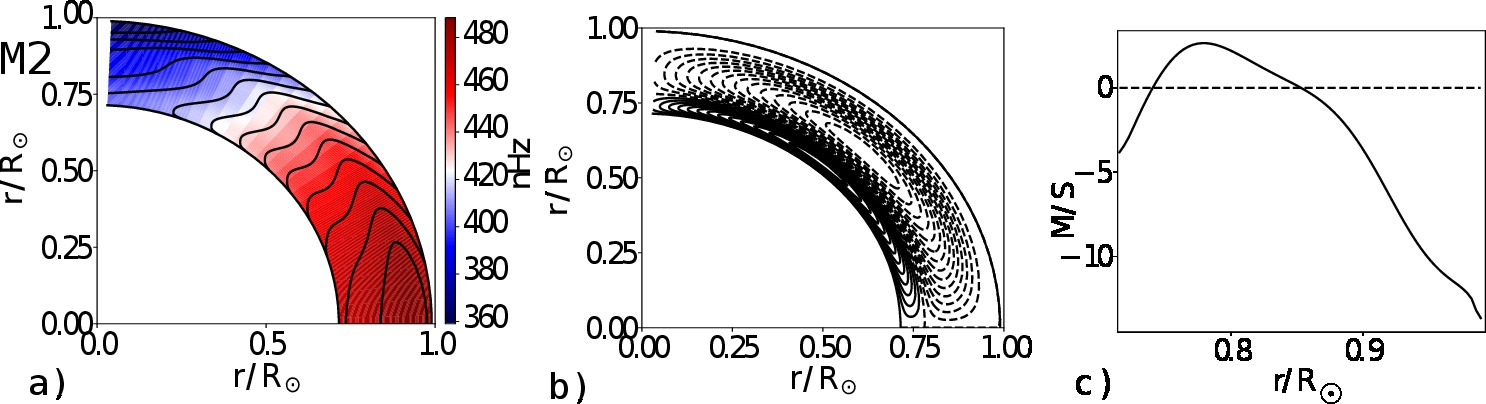# 91. On The Origin of the Double-Cell Meridional Circulation in the Solar Convection Zone

Contributed by Valery Pipin. Posted on March 19, 2018

V. V. Pipin1,2 and A. G. Kosovichev3,4,5
1 National Astronomical Observatory of Japan, 2-21-1 Osawa, Mitaka, Tokyo 181-8588, Japan
2 Institute of Solar-Terrestrial Physics, Russian Academy of Sciences, Irkutsk, 664033, Russia
3 Center for Computational Heliophysics, New Jersey Institute of Technology, Newark, NJ 07102, USA
4 Department of Physics, New Jersey Institute of Technology, Newark, NJ 07102, USA
5 NASA Ames Research Center, Moffett Field, CA 94035, USA

Abstract
According to the mean-field theory the double-cell circulation pattern can result from the sign inversion of a non-diffusive part of the radial angular momentum transport (the so-called Λ-effect) in the lower part of the solar convection zone. Here, we show that this phenomenon results from the radial inhomogeneity of the Coriolis number which depends on the convective turnover time. We demonstrate that if this effect is taken into account, solar-like differential rotation and double-cell meridional circulation can both be reproduced by the mean-field model. The model is consistent with the distribution of turbulent velocity correlations determined from observations by tracing motions of sunspots and large-scale magnetic fields, which indicate that these tracers are rooted just below the shear layer.

Introduction
The origin of the solar meridional circulation is related with the origin of the differential rotation. The two components of the global flow in the solar convection zone are dynamically linked via the centrifugal and baroclinic forces and because of the angular momentum transport by means of the meridional flow. The solar differential rotation can be generated by the nondissipative angular momentum flux in a rotating convection zone, or in other words, by the so-called Λ-effect. Figure 1 gives the simplified scenario of the Λ-effect in the Northern hemisphere of the Sun. At some level of the convection zone, the direction of the mean angular momentum transport is determined by the balance of the retrograde and accelerating torques from the rising and falling convective blobs:$\Omega\sin\theta \frac{\big(\big(\tau_c\frac{l}{\bar{\rho}}\frac{\partial \bar{\rho}}{\partial r}\big)_R - \big(\tau_c\frac{l}{\bar{\rho}}\frac{\partial \bar{\rho}}{\partial r}\big)_F\big)}{\Delta r} \sim \Omega\sin\theta\big(\frac{\partial}{\partial r} \frac{\tau_c l}{\bar{\rho}}\frac{\partial \bar{\rho}}{\partial r} \big)$
Therefore, the origin of the Λ effect is connected with effects of the Coriolis force and the density stratification. These two physical factors are quantified by the typical convective turnover time τc and the density stratification scale. If we assume that τc is constant over the depths then the sign of the radial angular momentum transport is determined by the density stratification. In this case the angular momentum transport is inward.Figure 1| On the origin of the Λ effect, the updated ideas of Ref 1.

Result
Ref. 2 has shown that with an accurate description of the angular momentum transfer, the Λ effect reverses signs in the depth of the convection zone. This results in a double-cell meridional circulation structure. The results for our complete model (with the sign reversal of the Λ- effect and convective anisotropy) are presented in Figure 2. The differential rotation profile shows a subsurface shear layer, which is roughly in agreement with the helioseismology inversion results. The model shows the double-cell meridional circulation structure, and the upper meridional circulation cell has its stagnation point at r = 0.87R, close to results of Ref. 3.Figure 2| (a) Distribution of the rotation rate,$\Omega(r,\theta) / 2\pi$, in the solar convection zone; the isolines are plotted in the range of 385-480 nHz with an interval of about 7nHz. (b) Streamlines of the meridional circulation. (c) Radial profile of the meridional velocity component at$\theta = 45^\circ$.

In summary, the double-cell meridional circulation on the Sun is naturally explained in our model because of a concurrent effect of the density stratification and variations of the Coriolis force on the cyclonic convection. The key point is that the variation of the convective turnover time with depth result in reversal of sign of the non-dissipative turbulent stresses (the Λ-effect) in the lower part of the convection zone. However, the properties of the turbulent angular momentum transport employed in this paper using the mean-field hydrodynamics approach require further studies with the help of observations and numerical simulations.

### References

 Kitchatinov, L. L. 1987, Geophys & Astrophys. Fluid Dynamics, 38, 273
 Pipin, V. V., & Kosovichev, A. G. 2018, ApJ, 854, 67
 Zhao, J., Bogart, R. S., Kosovichev, A. G., Duvall, Jr., T. L., & Hartlep, T. 2013, ApJL, 774, L29